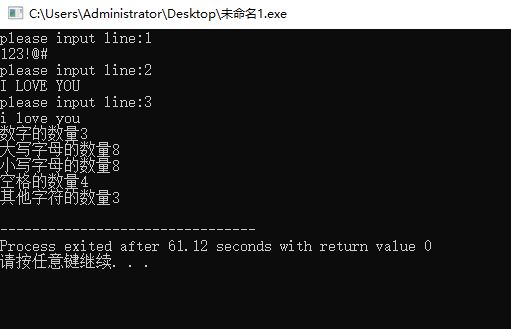• C语言实现判断一个字符串中标点符号的个数
2021-12-05 17:33:46
#include <stdio.h>
#include <ctype.h>
int main()
{
//C语言实现判断一个字符串中标点符号的个数
char *num=(char *)malloc(sizeof(char)*1000);  //具体大小视情况而定
scanf("%[^\n]",num);     //输入字符串，知道\n为止
int x=0;
while(*num)       //从字符串首地址开始取值，只要为标点就继续循环
{
if(ispunct(*num))   //这个函数需要调用#include<ctype.h>,用来记录标点的个数
x++;
num++;
}
printf("%d",x);   //打印个数
return 0;
}


更多相关内容
• 要求分别统计出其中英文大写字母，小写字母，数字，空格以及其他字符的个数。 分析：从题目中可以看出需要区分的有英文的大写字母和小写字母、数字以及空格或其他特殊符号。 #include<stdio.h> int main() { ...

## 有一篇文章，共有三行文字，每行有80个字符。要求分别统计出其中英文大写字母，小写字母，数字，空格以及其他字符的个数。

（1）分析：从题目中可以看出需要区分的有英文的大写字母和小写字母、数字以及空格或其他特殊符号。

#include<stdio.h>
int main()
{

int i,j,bigletter=0,smallletter=0,number=0,space=0,other=0;//行数和字符数的定义，各个字符数目的定义
char a;
for(i=0;i<3;i++)
{
gets(a[i]);
for(j=0;j<80&&a[i][j]!='\0';j++)
{
if(a[i][j]<='z'&&a[i][j]>='a')
smallletter++;
else if(a[i][j]<='Z'&&a[i][j]>='A')
bigletter++;
else if(a[i][j]<='9'&&a[i][j]>='0')
number++;
else if(a[i][j]==' ')
space++;
else
other++;
}
}
printf("数字的数量：%d\n大写字母的数量：%d\n小写字母的数量：%d\n空格的数量：%d\n其他字符的数量：%d\n",number,bigletter,smallletter,space,other);
/*printf("数字的数量:%d\n");
putchar(number);
printf("大写字母的数量:%d\n");
putchar(bigletter);
printf("小写字母的数量:%d\n");
putchar(smallletter);
printf("空格的数量:%d\n");
putchar(space);
printf("其他字符的数量:%d\n");
putchar(other);
//对应于开头的输入get a[i],我们可以想到输出的putchar，结果也是一样的
*/
return 0;
}



（2）输入一些句子之后，可以看到运行的结果是正确的展开全文• C语言实现统计字符个数#includeint main(){int sz={0},zm={0},z={0},i,space=0,e=0,t=0;char c;printf("请输入一段字符，统计其中各字符的数量\n");while((c=getchar())!='\n'){if(c<='z'&&c...

C语言实现统计字符个数

#include

int main()

{

int sz={0},zm={0},z={0},i,space=0,e=0,t=0;

char c;

printf("请输入一段字符，统计其中各字符的数量\n");

while((c=getchar())!='\n')

{

if(c<='z'&&c>='a')

zm[c-'a']++;

else if(c<='Z'&&c>='A')

z[c-'A']++;

else if(c<='9'&&c>='0')

sz[c-'0']++;

else if(c==' ')

space++;

else

e++;

}

printf("\n\n");

for(i=0;i<=9;i++)

{

t++;

printf(" %d的个数为%d ",i,sz[i]);

if(t%3==0)

printf("\n");

}

t=0;

printf("\n\n\n");

for(i=0;i<=25;i++)

{

t++;

printf(" %c的个数为%d ",i+97,zm[i]);

if(t%3==0)

printf("\n");

}

t=0;

printf("\n\n\n");

for(i=0;i<=25;i++)

{

t++;

printf(" %c的个数为%d ",i+65,z[i]);

if(t%3==0)

printf("\n");

}

t=0;

printf("\n\n\n");

printf(" 空格的个数为%d\n\n",space);

printf(" 其他字符的个数为%d\n",e);

return 0;

}

再来一则C语言统计输入字符个数的代码

#include

#define MAXWORD 30 //单词的最大长度

#define IN 1 //在单词内，即没有遇到空格

#define OUT 0 //在单词外，即遇到了空格

/*word length ==> wl单词长度简称*/

int wl()

{

char c;

int i;

int nc;

int state; //state为单词的状态：IN或OUT;

int overflow; //操过MAXWORD的单词个数

int wl[MAXWORD]; //长度为1~30的的字符长度统计

state = OUT;

nc = 0;

overflow = 0;

for(i = 1; i < MAXWORD; ++i)

wl[i] = 0;

while((c = getchar()) != EOF)

if(c == ' ' || c == '\n' || c == '\t')

{

state = OUT;

if(nc > 0)

if(nc < MAXWORD)

++wl[nc];

else

++overflow;

nc = 0;

}

else if(state == OUT)

{

state = IN;

nc = 1;

}

else

++nc;

for(i = 1; i < MAXWORD; ++i)

printf("长度为：%d的单词个数为：%d : \n\n", i, wl[i]);

return 0;

}

main()

{

wl();

}

以上所述就是本文的全部内容了，希望大家能够喜欢

展开全文• import feedparser # package to parse rssimport re # package to match regular expressiondef getwordscount(url):d = feedparser.parse(url)sum = 0 # sum for all the wordssum_en = 0sum_zh = 0sum_punc = 0wo...

import feedparser # package to parse rss

import re # package to match regular expression

def getwordscount(url):

d = feedparser.parse(url)

sum = 0 # sum for all the words

sum_en = 0

sum_zh = 0

sum_punc = 0

wordcount_zh = {} # dict to store chinese and its frequency

wordcount_en = {}

wordcount_punc = {}

for e in d[‘entries‘]:

if ‘summary‘ in e:# every entry should have a ‘summary‘ or a ‘description‘

summary = e.summary

else:

summary = e.description

words_zh, words_en, words_punc = getwords(e.title + ‘ ‘ + summary)

for word in words_zh:

wordcount_zh.setdefault(word, 0)#to set a dict[item] to default 0 if it doesn‘t exist

wordcount_zh[word] += 1

sum_zh += 1

for word in words_en:

wordcount_en.setdefault(word, 0)

wordcount_en[word] += 1

sum_en += 1

for word in words_punc:

wordcount_punc.setdefault(word, 0)

wordcount_punc[word] += 1

sum_punc += 1

sum = sum_zh + sum_en + sum_punc

wordcount_zh = sorted(wordcount_zh.items(), key = lambda d:d, reverse = True)# sort the dict with the value, and from the largest to smallest

wordcount_en = sorted(wordcount_en.items(), key = lambda d:d, reverse = True)

wordcount_punc = sorted(wordcount_punc.items(), key = lambda d:d, reverse = True)

print (d[‘feed‘][‘title‘])

print (‘Chinese: %d

%s‘%(sum_zh, wordcount_zh))

print (‘English: %d

%s‘%(sum_en, wordcount_en))

print (‘Punctuation: %d

%s‘%(sum_punc, wordcount_punc))

print (‘There are %d words in total‘%(sum))

def getwords(html):

txt = re.compile(r‘]+>‘).sub(‘ ‘, html)# re to replace ‘

‘ and so on with backspace

words_zh = re.compile(r‘[u4E00-u9FFA]‘).findall(txt)# chinese character is between u4E00-u9FFA

words_en = re.compile(r‘[A-Za-z]+‘).findall(txt)

words_punc = re.compile(r‘[s+.!/_,\$%^*(+"‘]+|[+——！，。？、[email protected]#￥%……&*()]+‘).findall(txt)

return words_zh, words_en, words_punc

展开全文• 统计单词个数，如何去掉单词后面的标点符号统计一个txt文本中英语单词的个数，用的fscanf函数，总是把单词后面的逗号统计进去了，请教各位高手如何去掉标点符号呢？代码如下：voidmain(){chars={0};inti=...
• 满意答案GUYE1172013.04.26采纳率：40%等级：11已帮助：3664人...逗号 0x2c 在 b[0x2c-0x20]分号 0x3b 在 b[0x3b-0x20]其它 标点符号 请查 ASCII 码，存 b[ASCII - 0x20].字符总数 n.----------------------------...
• c语言程序设计 输入一行字符，统计其中单词的个数。各单词之间用空格、标点符号分隔，空格、标点符号 可以是多个。例如输入：He_is__boy.”, 输出：“3”。
• 你这个不能返回三个值的 } printf("大写字母个数：%d\n小写字母个数：%d\n标点符号个数：%d\n", a, bi, c); //这个要放在循环外面的 } int main() //标准的写法,void main()是上世纪淘汰的古老写法 { char t;...
• #include #include using namespace std; int main(int argc, char *argv[]) { string s("hello world!!!"); decltype(s.size()) punct_cnt=0; for(auto c:s) if(ispunct(c)) ++CC++
• 本文给出计算一个字符串中单词的个数的C语言程序，感性趣的朋友可以看看。
• def replace_all_blank(value): 去除value中的所有非字母内容，包括标点符号、空格、换行、下划线等 :param value: 需要处理的内容 :return:返回处理后的内容 # w 表示匹配非数字字母下划线 result =re.sub(w+, , ...
• 1、先去除文件标点符号并且把大写改成小写。 #include #include #include #define MAX 30 typedef struct node {  char s;  struct node* next;  int ...
• 本题目要求编写程序统计一行字符中单词的个数。所谓“单词”是指连续不含空格的字符串，各单词之间用空格分隔，空格数可以是多个。 输入格式: 输入给出一行字符。 输出格式: 在一行中输出单词个数。 输入样例: ...
• 统计一行字符（包含空格、标点符号）的单词个数 //统计输入的字符串中有多少个单词 char s; int i=0,count=0; gets(s); int len = (int)strlen(s); printf("length=%d\n",len); int flag=0; char c; for...
• 具体代码如下所述:#...//num 用来统计单词的个数//state 用来记录程序当前是否处于一个单词之中，初值为0，表示不在单词中，值为1，表示正处于在一个单词中printf("Please input the number of lines for Engli...
• 摘要：字数统计是文档处理中经常要用到的功能，这其中要包括英文单词、数字和中文等，而且又要和全角或半角的标点符号进行区分，故此在中英文混合文件中进行准确的字数统计也是有一定难度的。这里提出了一种较好的...
• C语言I博客作业02 这个作业属于哪个课程 https://bbs.csdn.net/topics/602023653 这个作业要求在哪里 https://editor.csdn.net/md?not_checkout=1&articleId=120841732 这个作业的目标 <灵活运用...深度学习 python算法
• 输入一行字符，分别统计出其中英文字母、空格、数字和其他字符的个数。 输出格式为："英文字母=%d, 空格=%d, 数字字符=%d, 其它字符=%d". 注意：其中的逗号"，"为中文标点符号
• ## 统计文章单词频数(C语言)

千次阅读 多人点赞 2020-04-11 17:26:49
统计文章单词频数一、引言1.1题目概述1.2题目分析二、解题过程2.1 单词存储2.2 分割字符串2.3 存放单词2.4 排序三、完整代码 一、引言 最近花了很多时间来处理老师给我们布置的这道作业题，感觉学习编程以来算是第一...字符串
• #include<iostream> using namespace std; int main() { int i,j; char a; for(i=0;i<3;i++){ gets(a[i]); } int x=0,d=0,k=0,b=0; for(i=0;i<3;i++){ for(j=0;j<....
• #include #include #include #include void wnz1(); void wnz2();...但可以输入字母和数字可以统计出来 但我用gets(str); fputs(str,fp); 又什么都不能输入，直接进入了下一个程序　奇怪 就是我封的那一个
• 7-11 字符串19：单词统计 (50分) 题目： 在这里插入代码输入一行英文句子，统计其中的单词个数(这里的单词是指有>=1个大小写字母连续出现所组成的子串，非大小写字母出现可认为一个单词结束。句子长度不超过50。 ...字符串
• 输入字符串，包含：字母、数字、标点符号，以及空格字符，并将其逆序输出。 例如，当输入字符串为“I am a student.”,输出为“.tneduts a ma I”，假设字符数组最大长度为30。 输入提示信息：“Please Enter String...字符串
• 文本文件单词统计C语言） 本人目前还是一位在校大学生，写文章的目的是为了记录一下当前所学，与其他爱好者或从业者相互学习交流。文本文件单词统计这个题目是学校的一次课程设计中的一道，在编写时也是参考了很多...数据结构 字符串 算法
• 习题6-1 分类统计字符个数 (15分) 本题要求实现一个函数，统计给定字符串中英文字母、空格或回车、数字字符和其他字符的个数。 裁判测试样例： #include <stdio.h> #define MAXS 15 void StringCount( char ...字符串 数据结构
• # 所有十六进制数字字符 #输出：'0123456789abcdefABCDEF' print(string.octaldigits) # 所有八进制数字字符 #输出：'01234567' print(string.punctuation) # C语言环境中被认为是标点符号的所有字符 #输出：'!...
• 文本读入能够分离出单词，过滤数字和标点符号，把字母大小写转为小写，按英文字母的顺序高招二叉树，遍历但不递归；用单链表存储结构，对两个集合的内容进行并，交，差集运算...